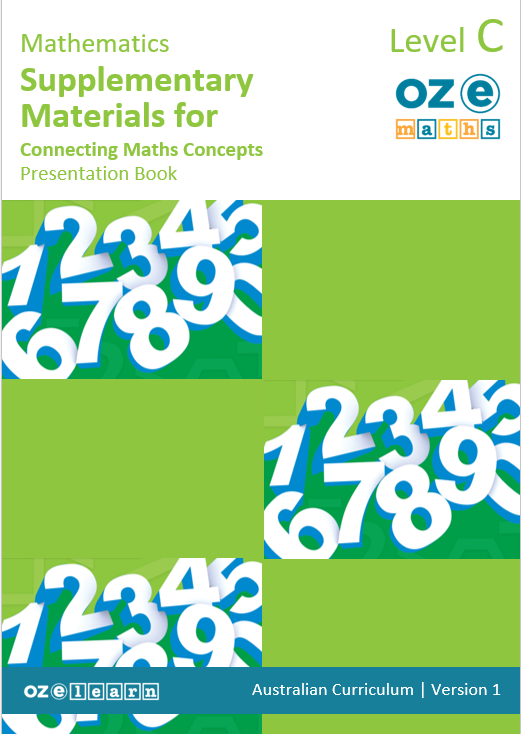## Oz-e-maths Supplementary Materials for Connecting Maths Concepts Years F-5

Top-ups and Swap-outs for Currency and Measurement – Year 5### Overview

Top-ups and Swap-outs for Currency and Measurement – Year 5 is a Mathematics unit for Year 5 students. It works in cooperation with Connecting Maths Concepts (CMC) to ensure that delivery of CMC aligns with Australian Curriculum gap requirements. It aligns to the Australian Curriculum:

• Select and use appropriate displays or visualisations, interpret and communicate findings or solutions within the context (AC9M5ST03).
• Justify choices and explain results in terms of the situation (AC9M5N09).
• The outcome in this lesson is now a Year 6 outcome
• Use observation and experience to assign probabilities that events occur in a given context, using fractions, percentages and decimals to indicate their estimated likelihood (AC9M6P01)
• Recognise and describe what changes and what remains the same under the transformation and identify any rotational symmetries (AC9M5SP03)
• Create simple financial plans (ACMNA106)
Success criteria
Top-Ups
• Apply directional language and understand the compass points
• Collect and identify categorical and numerical data types
• Understand budgeting
• Define probability using language and fractions
• Understand symmetry, transformations, translations, reflections, or rotations of two-dimensional shapes
Swap-Outs
• Apply arithmetic skills for all units of measurement
• To solve comparison word problems
Learning objectives
In Lesson 20 (CMC Level F: Lesson 31-40) students learn to:
• Use the grid reference system to describe location.
• Apply directional language using the compass points (North, East, South and West).
• Use a legend to identify landmarks on a map.
• Construct a legend from the information contained in a map, including a compass.
In lesson 21 (CMC Level F: Lessons 41-50) students learn to:
• Define data and how it can be displayed.
• Examine data question types.
• Identify and use open questions to closed questions.
• Identify categorical and numerical data types.
• Collect categorical data and conduct a survey.
In Lesson 23 (CMC Level F: Lesson 61-70) students learn to:
• Calculate a tuckshop order.
• Define and calculate a budget.
• Calculate a budget.
• Create a budget for a fundraising event.
• Create a budget for a class fundraising event.
• Define and identify GST on items.
In Lesson 25 (CMC Level F: Lesson 81-90) students learn to:
• Define probability - certain, likely, even chance, unlikely and impossible - and use these terms to describe the probability of an event occurring.
• Describe the probability of events occurring using certain, likely, even chance, unlikely and impossible.
• Recognise that probabilities can be expressed as a fraction from 0 to 1.
In Lesson 28 (CMC Level F: Lesson 111-120) students learn to:
• Define and identify the rotational symmetry of two-dimensional shapes.
• Identify the points of rotational symmetry.
• Identify rotational symmetry in patterns.
• Identify reflection symmetry using the x or y axis to complete the shape.
• Identify line symmetry by drawing a reflection.
• Define translation and use this term to describe two-dimensional shapes.
• Use translation to describe two-dimensional shapes.
• Describe the translations, reflections, or rotations of two-dimensional shapes.
• Use rotational symmetry to draw a two-dimensional shape.
• Define transformation and identify translations, reflections, and rotations.
• Use enlargement transformation to draw two-dimensional shapes.
Assessment
Mastery Test
Mastery Tests are given at the end of every 10 lessons, designed to be taught over a two-week cycle. Mastery Tests allow teachers to monitor student understanding of the concepts taught in previous lessons and to identify where reteaching is needed. The Teaching Guide contains the Mastery Test script. Mastery Tests are delivered using a presentation script, board displays and a handout for students. The Teaching Guide also contains the Answer Key.

Cumulative Tests
There are two Cumulative Tests for Swap-Outs that are completed after lesson 60 and lesson 120. The Cumulative Tests are in the Teaching Guide. Teachers copy the assessment and distribute to students at testing time.

## Recommended Units

\Learnworlds\Codeneurons\Pages\ZoneRenderers\CourseCards